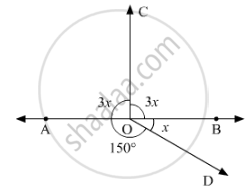Share
Notifications

View all notifications
Books Shortlist
Your shortlist is empty

# In Below Fig, Determine the Value of X. - CBSE Class 9 - Mathematics

Login
Create free account

Forgot password?
ConceptConcept to Lines and Angles

#### Question

In below fig, determine the value of x.#### Solution 1

In the given figure:AOB is a straight line. Thus,∠AODand  ∠BODform a linear pair.

Therefore their sum must be equal to180°.

We can say that

∠AOD+∠BOD = 180°

It is given that ∠AOD=150°, substituting this value in equation above, we get:

150° + x = 180°

 x = 180° - 150°

  x =30°

#### Solution 2

Since sum of all the angles round a point is equal to 360°.Therefore

⇒ 3x + 3x +150 + x = 360°

⇒ 7x° = 360° -150°

⇒ 7x = 210°

⇒ x = 210/7

⇒ x = 30°

Is there an error in this question or solution?

#### APPEARS IN

Solution In Below Fig, Determine the Value of X. Concept: Concept to Lines and Angles.
S